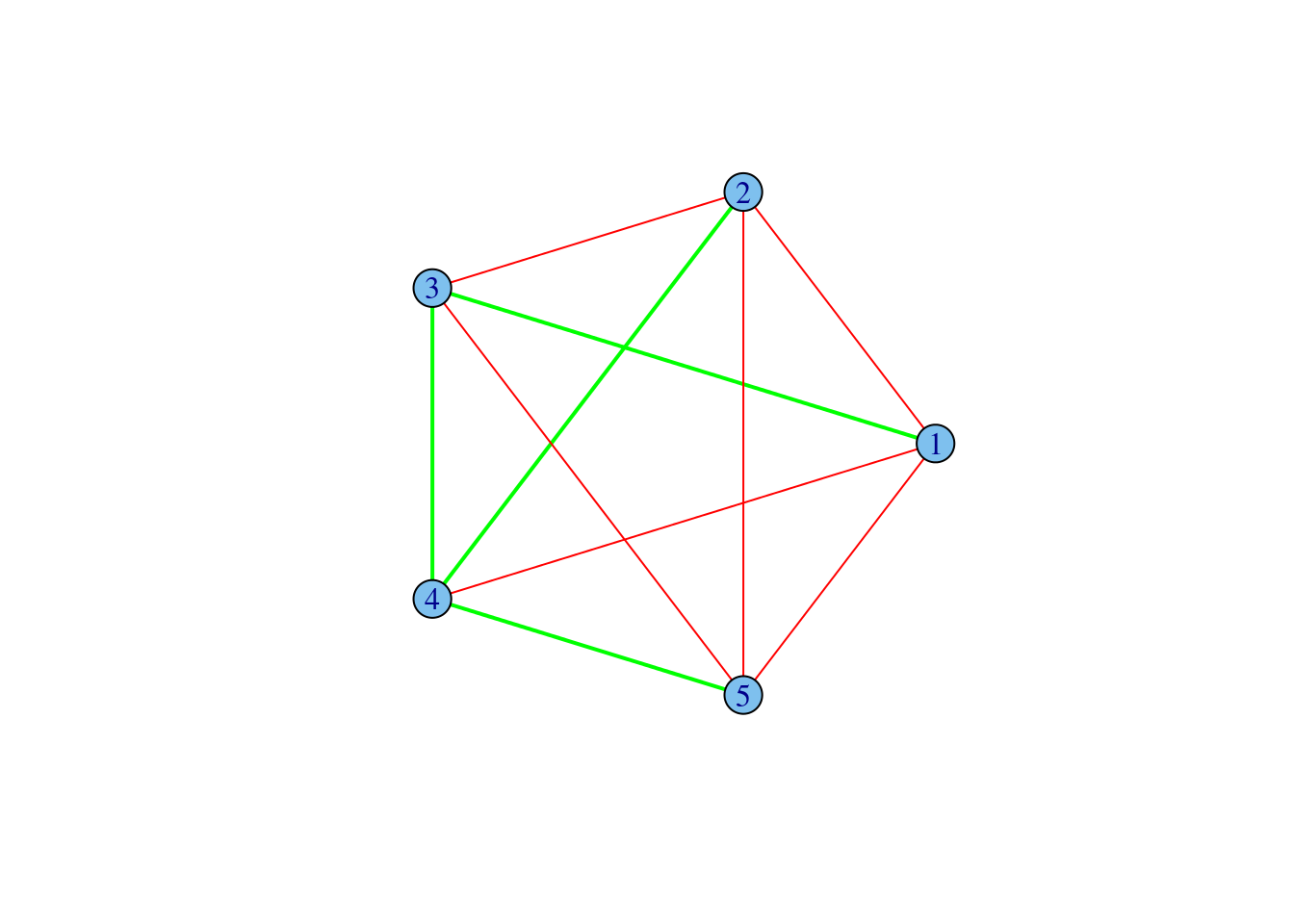## Installation

For installation Igraph package, you should just fire up an R shell and type the following command:

Next, R gives you some information on the installation of the package. It may be something like this:

Installing package(s) into ‘D:/R/library’(as ‘lib’ is unspecified) …. opened URL

package ‘fortunes’ successfully unpacked and MD5 sums checked

….

# First steps

Before you can use a package, you have to load it into R by using the $$library(...)$$ function.

library(igraph)

You should load library at the beginning of each session. To start use the package you also should learn the basics of graph theory (or basic definitions). You can find them on http://en.wikipedia.org/wiki/Graph_%28mathematics%29.

## CREATING GRAPHS

There are many functions to create different graph structures in Igraph. Some of them you can find below. Since you can create one of two types of graph (directed and undirected), to avoid confusion, it is better to explicitly specify which type you are creating (directed=TRUE or directed = FALSE).

### Graph structures:

• Empty graphs (a set of disconnected vertices)
g<-graph.empty(n=10, directed=TRUE)

Complete graph (each pair of vertices if this graph has an edge connecting them, the argument LOOPS = FALSE means, that self edges are not added)

• Complete graph
g<-graph.full(n=10, directed = FALSE, loops = FALSE)
• Stars
g<-graph.star(n=10, mode="out")

g<-graph.star(n=10, mode="in")
• Rings
g<-graph.ring(n=10)
• Graphs with the given list of edges
edges <- c(1,2, 3,2, 2,4)
g<-graph(edges, n=max(edges), directed=TRUE)

See more examples at http://igraph.org/r/doc/graph.constructors.html

## GAINING INFORMATION ABOUT GRAPH STRUCTURE

$$vcount$$ and $$ecount$$ return integer constants ? the number of vertex. $$neighbors$$ returns an integer vector ? the number of neighbors for the vertex. $$is.directed$$ and $$are.connected$$ return boolean constants. You can use these function to know the type of graph (directed or undirected) and to determine whether there is a link between vertices v1and v2 in graph $$.get.edgelist$$ function returns the list of edges in a graph. To obtain the adjacency matrix of a graph use $$get.adjacency$$ function (you also need to install package ?Matrix?in this case).

edges <- c(1,2, 3,2, 2,4)
g<-graph(edges, n=max(edges), directed=TRUE)
vcount(g)
##  4
ecount(g)
##  3
neighbors(g, V(g), mode = 1)
##  2
incident(g,V(g), mode=c("all", "out", "in", "total"))
##  3 1 2
is.directed(g)
##  TRUE
are.connected(g, V(g), V(g))
##  FALSE
get.edgelist(g)
##      [,1] [,2]
## [1,]    1    2
## [2,]    3    2
## [3,]    2    4

## GRAPH MODIFICATIONS

#### Adding attributes to vertices and edges:

Central concepts of igraph are vertex and edge sequences. For dealing with vertex and edges use functions for creating vertex and edges sequences:

V(g) # vertex sequence
## Vertex sequence:
##  1 2 3 4
E(g, P=NULL, path=NULL, directed=TRUE) # edge sequences
## Edge sequence:
##
##  1 -> 2
##  3 -> 2
##  2 -> 4

#### Adding new vertices and edges to the graph:

You can add some vertices or edges by using the following code:

add.edges(graph, edges, ..., attr=list())
add.vertices(graph, vertices, ..., attr=list())

## GRAPH VISUALISATION

Use function plot for visualization. This function has many parameters that allow you to get pretty picture of your graph. See more on http://igraph.org/r/doc/plot.common.html.

You also can save a graph in pdf.

pdf("Graph.pdf")
plot(graph)
dev.off()

## GRAPHS IMPORT

The $$read.graph$$ function is able to read graphs in various representations from a file, or from a http connection. More specifically the available formats read at http://igraph.org/r/doc/read.graph.html.

g <- read.graph("./graph.txt", format="edgelist")

g <- read.graph("./graph.dl", format="pajek")

Create graph from datatable:

advice_data_frame <- read.table('http://sna.stanford.edu/sna_R_labs/data/Krack-High-Tec-edgelist-Advice.txt')
g <- graph.data.frame(advice_data_frame)

dat=read.csv(file.choose(),header=TRUE,row.names=1,check.names=FALSE)
m=as.matrix(dat)
plot.igraph(net,vertex.label=V(net)$name,layout=layout.fruchterman.reingold, edge.arrow.size=0.5) ## GRAPHS EXPORT You can write your graph to file in various formats. write.graph(g, file='my_graph.dl', format="pajek") write.graph(g, file='my_graph.txt', format="edgelist") #### Examples: Create a ring graph with random number of vertex (between 5 and 10). How many edges and vertices in this graph? Print neighbors of the 5th vertex, the incident edges of this vertex. Are the 1st and the 3rd vertices connected? Are the 3rd and the 4th vertices also connected (disconnected)? To give answers for this question use special functions (do not plot the graph). n <-sample(5:10,1) g <- graph.ring(n) vcount(g) ##  6 ecount(g) ##  6 neighbors(g, 5) ##  4 6 incident(g, 5) ##  4 5 are.connected(g, 1, 3) ##  FALSE are.connected(g, 3, 4) ##  TRUE plot(g, layout = layout.fruchterman.reingold,vertex.label=V(g)$number,

edge.arrow.size=0.5)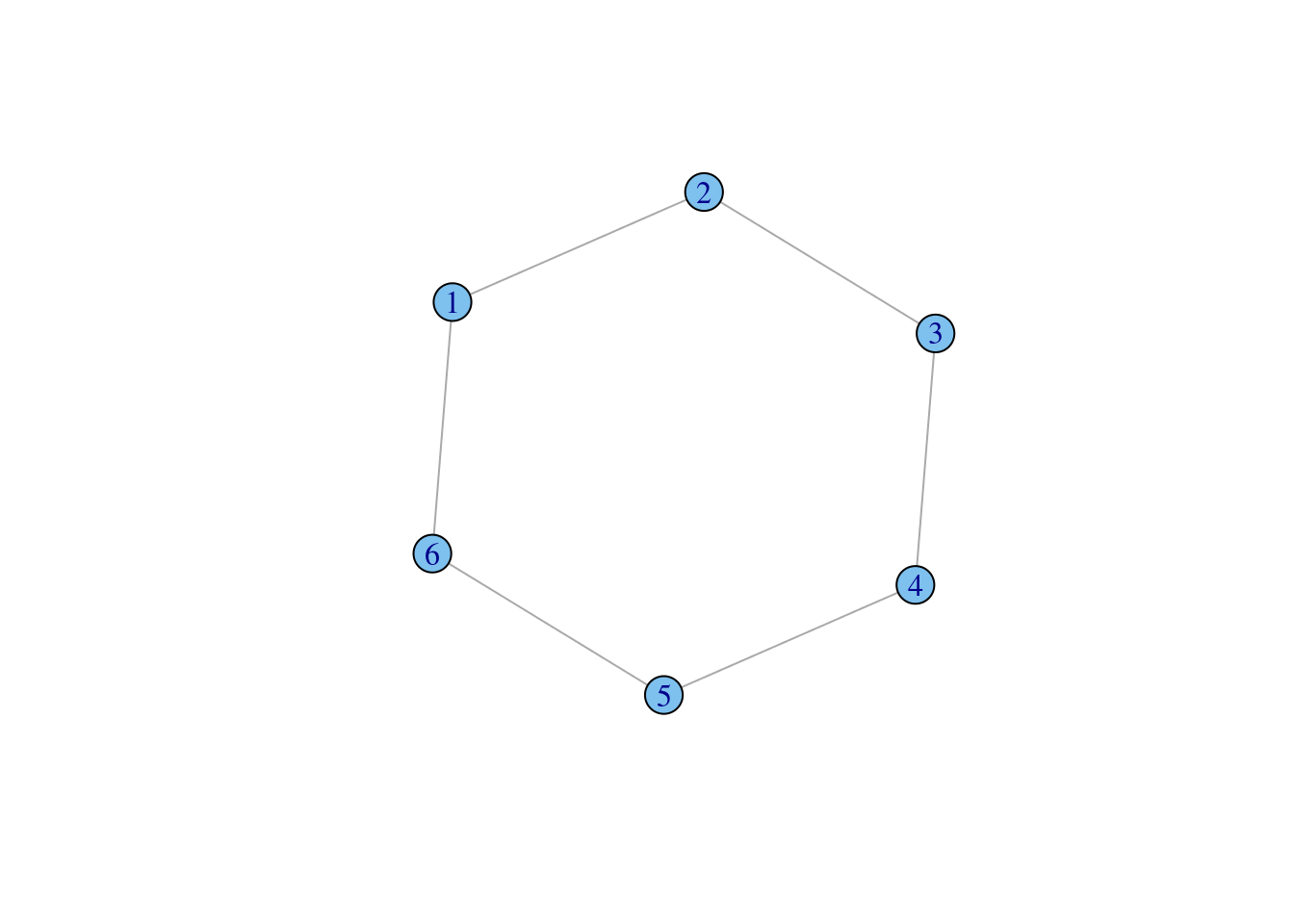Create an empty undirected graph with 5 vertices. Add edges between the following nodes: 1 and 3, 1 and 5, 2 and 5, 4 and 5. Plot this graph. Next, add the 6th vertex and connect it to that which has the most number of neighbors. Assign names to all vertices (for example, letters in alphabetical order) and some random weights to all of edges (it may be random values between 0 and 1). Print the adjacency matrix with weights.

g <- graph.empty (5, directed = FALSE)

new_edges <- c(1,3, 1,5, 2,5, 4,5)

plot(g)

V(g)$name <- letters[1:vcount(g)] E(g)$weight <- runif(ecount(g))

get.adjacency(g, attr="weight")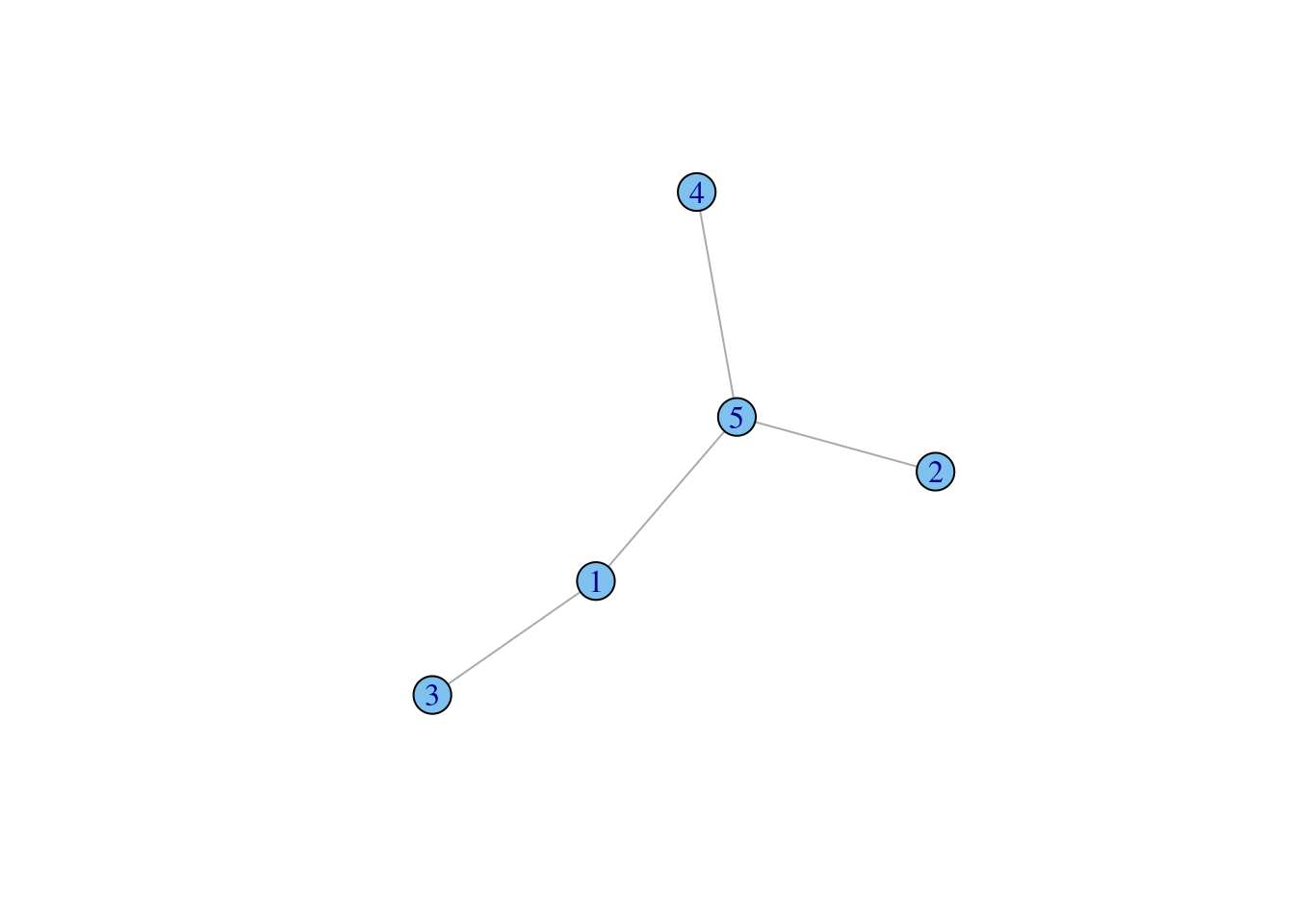## 6 x 6 sparse Matrix of class "dgCMatrix"
##           a         b         c           d           e         f
## a .         .         0.5977384 .           0.506598056 .
## b .         .         .         .           0.357016569 .
## c 0.5977384 .         .         .           .           .
## d .         .         .         .           0.007255013 .
## e 0.5065981 0.3570166 .         0.007255013 .           0.5647903
## f .         .         .         .           0.564790345 .
plot(g, layout = layout.fruchterman.reingold,vertex.label=V(g)$number)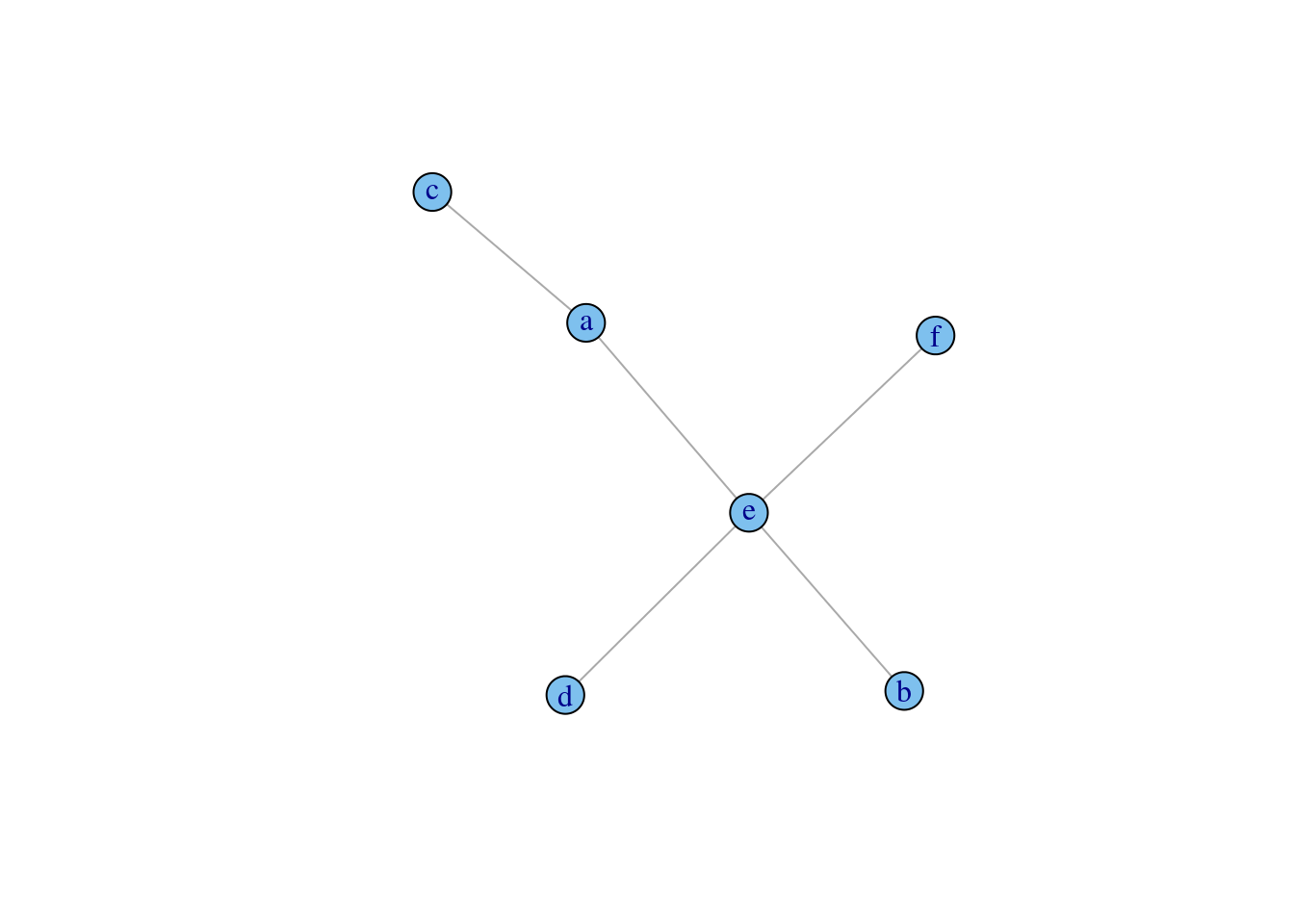Create a star graph with 8 edges. Next, assign random numbers between 1 and 50 to vertices and select vertices for which this value is less than 30, set the color of these vertices to green. g <- graph.star(8) V(g)$number <- sample(1:50, vcount(g), replace=TRUE)

V(g)$color <- "grey" V(g)[ number < 30 ]$color <- "green"

plot(g, layout=layout.circle, vertex.color=V(g)$color, vertex.label=V(g)$number)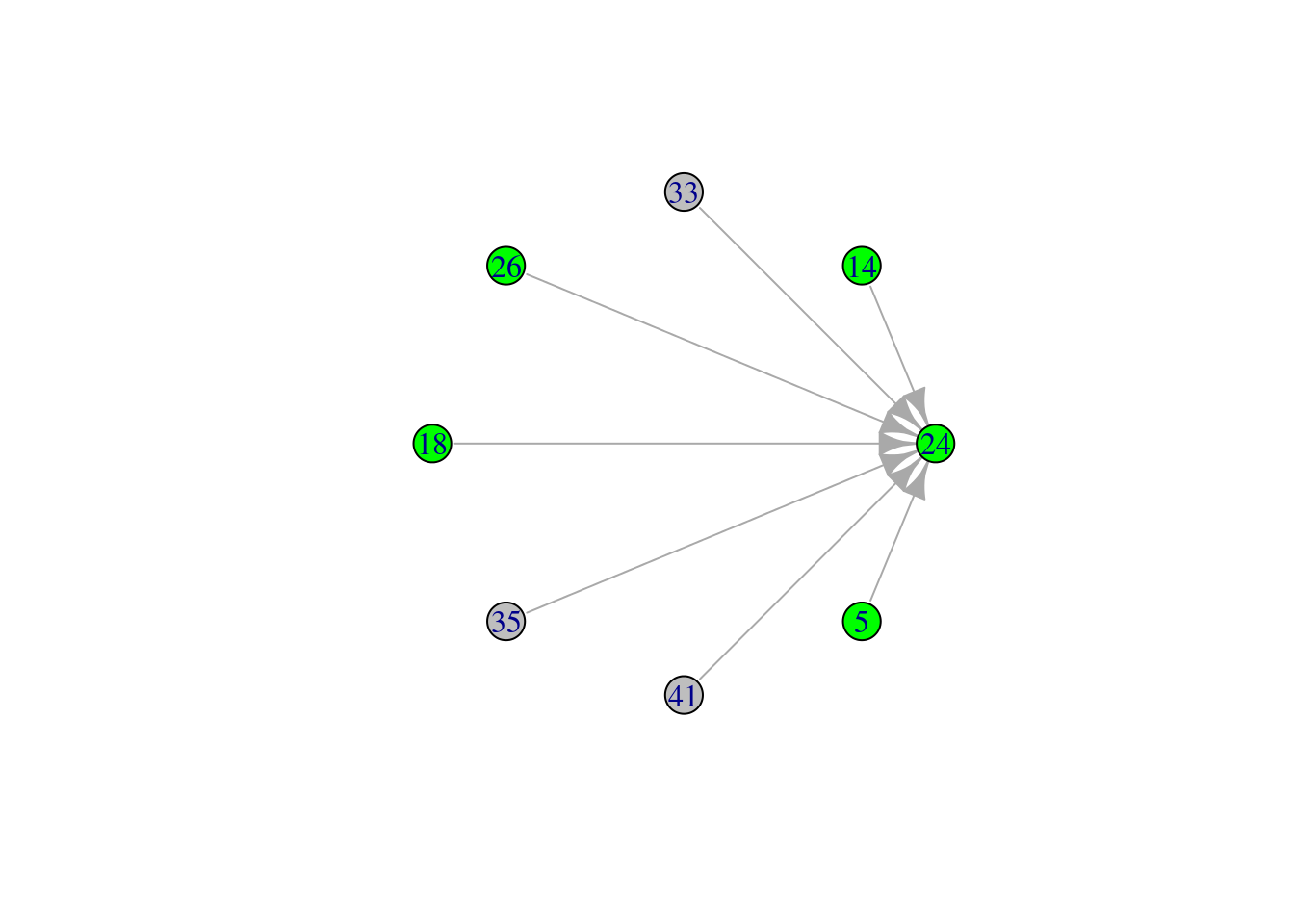Create a complete graph with 5 edges. Next, assign random weights between 0 and 1 to all of edges. Set width of edges to 2 and color to green for those of them that have weight less than 0.5, set the width value to 1, color to red for others.

g <- graph.full(5)

E(g)$weight <- runif(ecount(g)) E(g)$width <- 1

E(g)$color <- "red" E(g)[ weight < 0.5 ]$width <- 2

E(g)[ weight < 0.5 ]$color <- "green" plot(g, layout=layout.circle, edge.width=E(g)$width, edge.color= E(g)\$color)